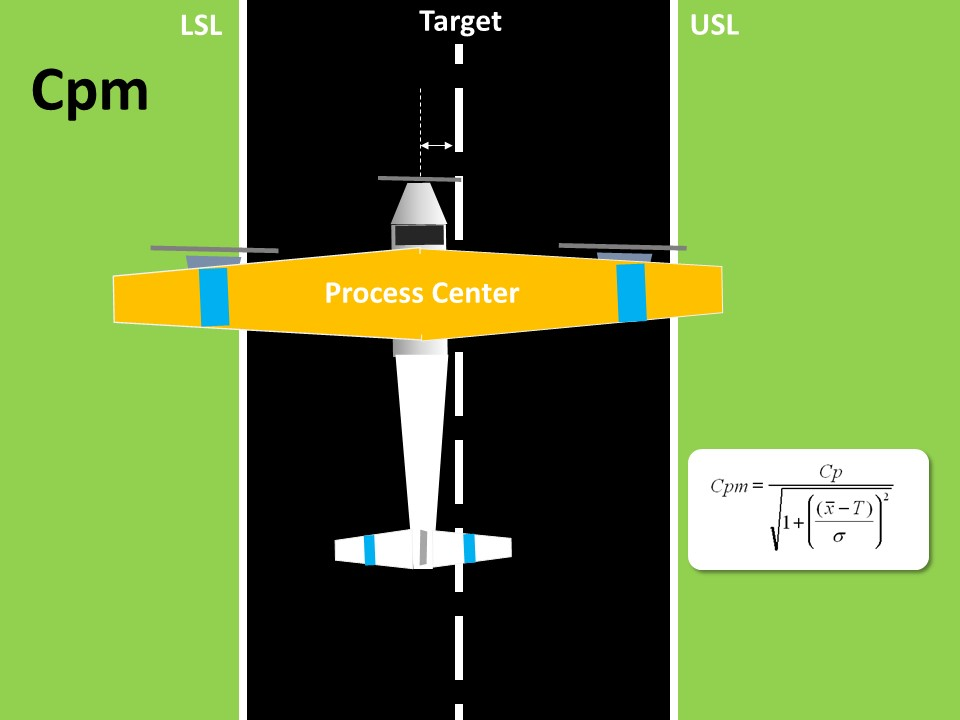Significance of Y=f(X) in Lean Six Sigma

If you are new to Lean Six Sigma then Y=f(X) is one amongst many jargons that you will have to familiarize yourself.
The objective of Lean Six Sigma philosophy and DMAIC improvement methodology is to identify the root causes to any problem and control/manage them so that the problem can be alleviated.
Six Sigma is process oriented approach which considers every task as a process. Even the simplest of the tasks, such as performing your morning workout or getting ready to office is considered as a process. The implication of such a view point is to identify what is the output of that process, its desired level of performance and what inputs are needed to produce the desired results.
Y is usually used to denote the Output and X for the inputs.
Y is also known as dependent variable as it is dependent on the Xs. Usually Y represents the symptoms or the effect of any problem.
On the other hand, X is known as independent variable as it is not dependent on Y or any other X. Usually Xs represents the problem itself or the cause.
As you will agree that any process will have at least one output but most likely to have several inputs. As managers, we all are expected to deliver results and achieve a new level of performance of the process such as Service Levels, Production Levels, Quality Levels, etc., or sustain the current level of performance.
In order to achieve this objective, we focus our efforts on the output performance measure.  However a smart process manager will focus on identifying Xs that impact the output performance measure in order to achieve the desired level of performance.
How does one identify the input performance measures or Xs?
Six Sigma DMAIC methodology aims to identify the inputs(Xs) that have significant impact on output (Y). After that the strength and nature of the relationship between Y and Xs are also established.
Six Sigma uses a variety of qualitative and quantitative tools & techniques listed below to identify the statistical validation of the inputs (or root causes), their strength and nature of relationship with Y:

• Cause and Effect Diagram/Fish Bone diagram
• 5 Why Analysis
• Process Maps
• Histogram
• Descriptive Statistics
• Run Charts
• Normal Distribution Plots
• Box plots
• Stem and Leaf Plots
• Hypothesis Testing
• ANOVA (Analysis of Variance)
• Chi-Square Test
• 1-t Test
• 2-t Test
• Paired t Test
• Correlation
• Regression
• Scatter Plots
• Statistical Process Control (SPC)/Control Charts

What does f in Y= f(X) mean?
‘f’ represents the nature and strength of the relationship that exists between Y and X. On one hand, this equation can be used for a generic interpretation that symbolizes the fact that Y is impacted by X and nature of relationship can be quantified. On the other hand, such a mathematical expression can be created provided we have sufficient data using the above mentioned analytical tools such as regression and other hypothesis tests.
The mathematical expression that we obtain is nothing but an equation such as:
TAT = 13.3 – 7.4*Waiting Time + 1.8*No. of Counters – 24*Time to Approve
Once such an equation is established, it can be easily used to proactively identify the Y for various values of X. Thus Y= f(X) is the basis for predictive modeling. All the newer analytical concepts such as Big Data, etc are based on this foundation principles.

Related ArticlesDifference between Cpk and CpmProtected: Data Sampling QuizCriteria for Successful Lean Six Sigma Project.